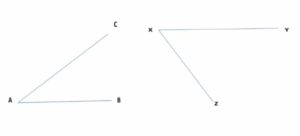# Lines, Angles and Bearing (Directions)

### LESSON – LINES, ANGLES AND BEARING

###### TOPIC
• Introduction
• Types of Lines
• Meaning and Types of Angles
• Bearing (Four Cardinal Points)
• Lesson Evaluation

###### INTRODUCTION

A line is a straight or curved mark made by marking materials like pen, pencil, etc.

A line can be thin or thick line. When two or more lines are combined, a shape, image or sign is formed.

Line is one of the important elements of design.

###### TYPES OF LINES

1. Vertical lines

2. Horizontal lines

3. Diagonal lines

4. Zigzag lines

5. Curved lines

6. Dotted lines

7. Dash lines

8. Parallel line

9. Perpendicular line

1. VERTICAL LINES

Vertical lines are straight lines that move up and down without any slant.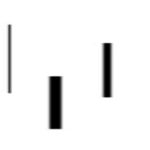2. HORIZONTAL LINES

Horizontal lines are straight lines that are parallel to the horizon.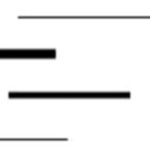3. DIAGONAL LINES

Diagonal lines are straight thin or thick lines that slant.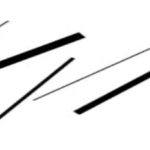4. ZIGZAG LINES

Zigzag lines are straight thin or thick lines made from a combination of diagonal lines.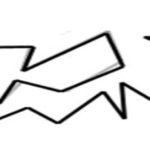5. CURVED LINES

Curved lines are thin or thick lines that change direction gradually.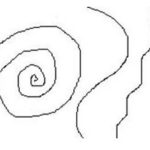6. DOTTED LINES

Dotted lines are lines made up of a series of dots.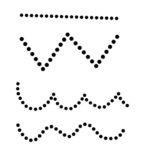7. DASH LINES

Dotted lines are lines made up of a series of dots.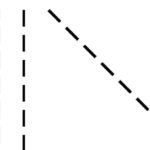8. PARALLEL LINES

Parallel lines are lines in a plane that are always the same distance apart like equal sign.

### ______________________

9. PERPENDICULAR LINES

Perpendicular lines are lines that intersect at right angles.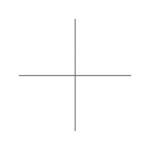###### USES/IMPORTANCE OF LINES

The uses of lines are as follows:

1. It is used to indicate a part.

2. It is used show direction.

3. It is used as a symbol.

4. It is used as a plan.

5. It is used to make a sketch.

6. It is used to mark out.

7. It is used to cut out a part.

8. It is used as measurement.

CLASS EXERCISE

1. Explain the meaning of lines.

2. state and draw types of lines.

3. differentiate between parallel and perpendicular lines.

4. what I’d the different between vertical and horizontal lines.

5. state 5 uses of lines.

###### CONCEPT OF ANGLE

An angle is the space between two intersecting lines.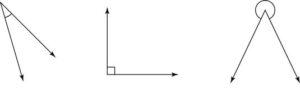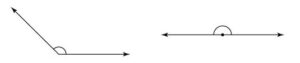###### TYPES OF ANGLES

An angle is the space between two intersecting lines.

There are different types of angles,

1. Right angle

2. Acute angle

3. Obtuse angle

4. Straight angle or angle on a straight line

5. Reflex angle

6. Angle on a point

7. Complimentary angle

8. Supplementary angle

1. RIGHT ANGLE

Right angle is the angle that forms a square corner.

It is an angle that is always equal 90°.

2. ACUTE ANGLE

Acute angle is any angle that less than 90°.

It is an angle between 1° – 90°.

Acute angle is greater 0° and less than 90°.

3. OBTUSE ANGLE

Obtuse angle is any angle that is larger (bigger) than right angle.

It is an angle between 91° – 179°.

Obtuse is greater than greater 90° and less than 180°.

4. STRAIGHT ANGLE

Straight angle is any angle that is equal to 180°.

It is twice of right angles.

Straight angle is equal to 180°.

5. REFLEX ANGLE

Reflex angle is any angle that is greater than 180° and less than 360°.

6. ANGLE AT A POINT

Angel at a point is any angle that is equal to 360°.

7. COMPLIMENTARY ANGLES

Complimentary angles are two angles that are sum up to 90º.

Two angles are said be complimentary angles, when they add up to 90°.

8. SUPPLEMENTARY ANGLE

Supplementary angles are two angles that are sum up to 180º.

Two angles are said be supplementary angles, when they add up to 180°.

###### CARDINAL POINTS

Bearing is the direction along which someone or something moves or faces.

It is used to describe or determine the position of someone or something.

Sometimes, it is used as right , left, forward (up) or backward (down).

Geographically, North, South, East and West are used in describing bearing.

These positions are known as cardinal points.

North, East, West and South are called primary points or positions while North East (NE), North West (NW), South East (SE) and South West (SW).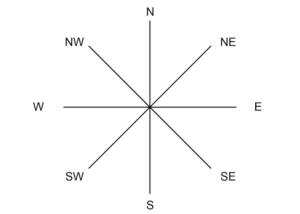###### ANGLES ON CARDINAL POINTS

The primary cardinal directions are North (0°), East (90°), South (180°) and West (270°) at 90° angles on the compass rose.

The secondary cardinal directions are formed by bisecting the primary cardinal directions to give North East (45°), South East (135°) and South West (225°), North West (315°).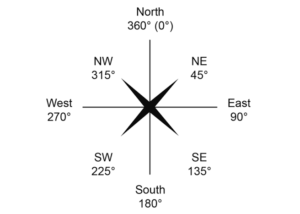WORKING EXAMPLES

Find the location of the following angles,

1. 60°

2. 390°

3. 80°

4. 120°

SOLUTIONS

1. NE

2. NE (390° – 360° = 30°)

3. NE

4. SE

1. 100°

2. 200°

3. 250°

4. 270°

5. 300°

6. 390°

7. 450°

8. 480°

9. 540°

10. 359°

###### WORKING EXERCISE 2

Draw and mark the following locations on cardinal points,

1. 135°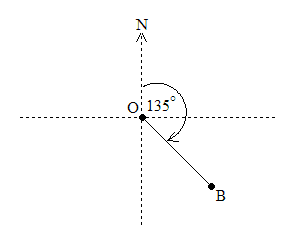2. 200°

3. 250°

4. 270°

5. 300°

6. 100°

###### WORKING EXERCISE 3

Give the complement of each of the following angles.

1. 51º

2. 13º

3. 46º

4. 65º

5. 53º

###### WORKING EXERCISE 4

Mention the supplement of each of the following angles.

1. 77º

2. 53

3. 67º

4. 32º

5. 48º

###### WORKING EXERCISE 5

Indicate it the sum is complementary or supplementary angles,

1. 114º + 66º =

2. 39º + 141º =

3. 68º + 22º =

4. 63º + 27º =

5. 94º + 86º =

6. 123º + 57º =

7. 41º + 49º =

8. 19º + 71º =

###### WORKING EXERCISE 6

Measure the following angles,